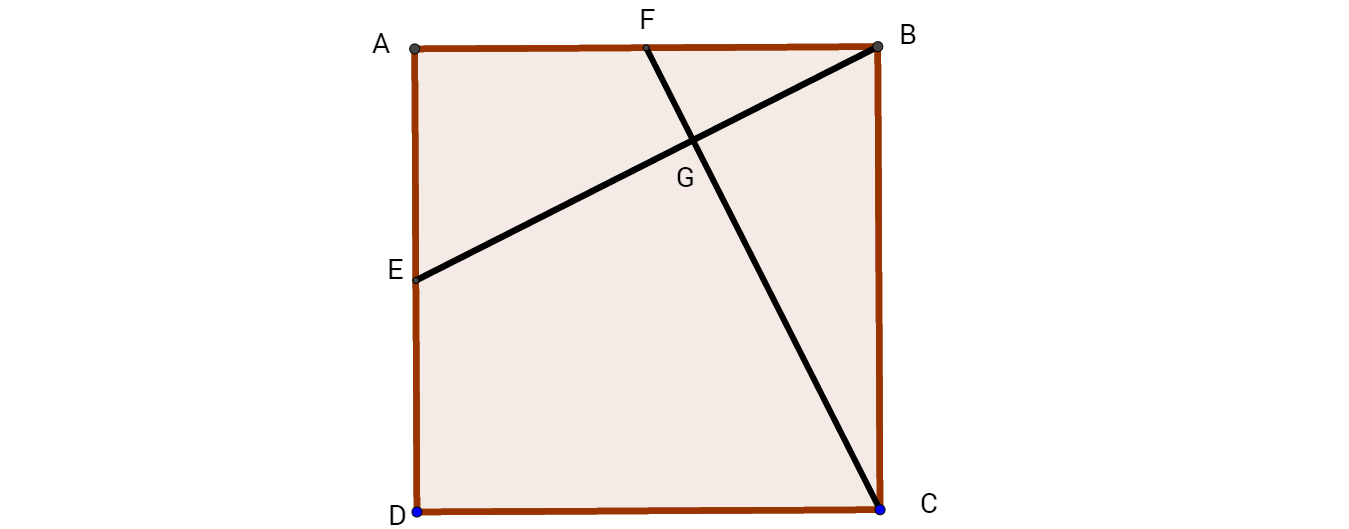# Square and 2016

Geometry Level 3$ABCD$ is a square with side length 2016. $E$ and $F$ are the mid-points of $AD$ and $AB$ respectively. $G$ is the intersection point of $CF$ and $BE$. Find the length of $DG$.

×